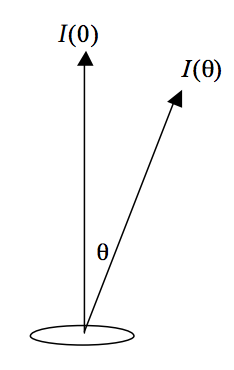$$\require{cancel}$$

# 1.13: Lambertian Surface

•• Contributed by Jeremy Tatum
• Emeritus Professor (Physics & Astronomy) at University of Victoria

A lambertian radiating surface [Johann Heinrich Lambert 1728 - 1777] is one whose intensity varies with angle according to Lambert's Law;

$I(\theta) = I(0) \cos \theta. \tag{1.13.1} \label{1.13.1}$$$\text{FIGURE I.2}$$

Consider a small element $$\delta A$$ of a lambertian radiating surface, such that the intensity radiated by this element in the normal direction is $$I(0)$$, and the normal radiance is therefore $$I (\theta )/\delta A$$. The radiance at angle $$\theta$$ is the intensity divided by the projected area:

$\frac{I(0) \cos \theta}{\delta A \cos \theta}= \frac{I(0)}{\delta A} \tag{1.13.2} \label{1.13.2}$

Thus the radiance of a lambertian radiating surface is independent of the angle from which it is viewed. Lambertian surfaces radiate isotropically. The radiance of a black body is lambertian. Since the Sun exhibits limb-darkening; the Sun is not a black body, nor is it lambertian.

For a reflecting surface to be lambertian, it is required that the radiance be independent not only of the angle from which it is viewed, but also of the angle from which it is irradiated (or illuminated). In discussing the properties of reflecting surfaces, one often distinguishes between two extreme cases. At the one hand is the perfectly diffusing lambertian surface; blotting paper is sometimes cited as a near lambertian example. The other extreme is the perfectly reflecting surface, or specular reflection (Latin speculum, a mirror), in which the angle of reflection equals the angle of incidence. It might be noted that expensive textbooks are often printed on specularly reflecting paper and are difficult to read, whereas inexpensive textbooks are often printed on paper that is approximately lambertian and are consequently easy to read.

The full description of the reflecting properties of a surface requires a bidirectional reflectance distribution function, which is a function of the direction ($$\theta_i$$ , $$\phi_i$$ ) of the incident light and the direction of the reflected (scattered) ($$\theta_r$$ , $$\phi_r$$) light. Also included in the theory are the several albedoes (normal, geometric and Bond). These concepts are of great importance in the study of planetary physics, but are not pursued further here. Some further details may be found, for example, in Lester, P. L., McCall, M. L. and Tatum, J. B., J. Roy. Astron. Soc. Can ., 73, 233 (1979).